Skip To Content

# Create and use a scatter plot

Scatter plots are used to determine the strength of a relationship between two numeric variables. The x-axis represents the independent variable, and the y-axis represents the dependent variable.

Scatter plots can answer questions about your data, such as: What is the relationship between two variables? How is it distributed? Where are the outliers?

## Examples

### Two variables

A public works department has noticed an increase in leaks on water mains. The department wants to know how much of an effect the total length of pipes has on the number of leaks versus the impact of properties of the pipes, such as age or circumference. A scatter plot can be used to plot the total number of leaks versus the total length of pipes in each zone.

The public works department also wants to know if there is any difference between pipes surveyed at different times of the year. Using the Color by option, you can style the points using unique colors for every unique value in the specified field.

The above scatter plot indicates that most of the pipe surveys occurred in April.

A scatter plot can use regression analysis to estimate the strength and direction of the relationship between dependent and independent variables. Statistical models are illustrated with a straight or curved line, depending on your selected chart statistic. The R2 value can be added to give a measure of the impact of the length of pipes on the number of leaks.

### Add a third variable

A public works department has noticed an increase in leaks on water mains. The department wants to know how much of an effect the total length of pipes has on the number of leaks versus the impact of properties of the pipes, such as age or circumference. The department also wants to know if there is a relationship between the number of leaks or length of pipes and the cost per day (including construction, maintenance and repairs, and lost resources through leaks). A scatter plot with proportional symbols can be used to plot the total number of leaks versus the total length of pipes in each zone, with the size of the points representing the cost per day.

##### Tip: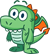Drag a number field to your page and drop it on your scatter plot to give your chart graduated symbols.

The public works department also wants to know if there is any difference between pipes surveyed at different times of the year. Using the Color by option, you can style the points using unique colors for every unique value in the specified field.

The above scatter plot indicates that most of the pipe surveys occurred in April.

## Create a scatter plot

To create a scatter plot, complete the following steps:

1. Select two number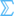or rate/ratio fields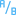.
2. Create the scatter plot using the following steps:
1. Drag the selected fields to a new card.
2. Hover over the Chart drop zone.
3. Drop the selected fields on Scatter Plot.
##### Tip:

You can also create charts using the Chart menu above the data pane or the Visualization type button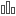on an existing card. For the Chart menu, only charts that are compatible with your data selection will be enabled. For the Visualization type menu, only compatible visualizations (including maps, charts, or tables) will be displayed.

Scatter plots can also be created using View Scatter Plot, which is accessed from the Action button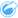under Find answers > How is it related?

## Usage notes

By default, scatter plots are symbolized using a single symbol. You can change the Chart Color using the Legend button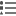. You can add a string field to the Color by variable on the x-axis to change the scatter plot to Unique symbols. If unique symbols are used, the legend can be used to select data on the scatter plot. To change the color associated with a category, click the symbol and choose a color from the palette or enter a hex value.

You can add a line of best fit to the scatter plot using the Chart Statistics button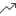. The line of best fit can be Linear, Exponential, or Polynomial. The equation of the line of best fit and the R2 value will also be displayed on the chart.

StatisticDescription

Linear

Linear regression attempts to fit a straight line through a set of values so that the distances between the values and the fitted line are as small as possible. A positively sloped line (from lower left to upper right of the chart) indicates a positive linear relationship. Positive relationships mean that values increase together. A negatively sloped line indicates a negative linear relationship. A negative relationship means that one value decreases as another increases. Goodness of fit measures, such as R2, can be used to quantify the relationship. The closer to 1, the stronger the relationship.

Exponential

This calculates an exponential (upward) curve of best fit to model a nonlinear relationship in your data (R2 at 0 or close to 0).

Polynomial

This calculates a curve of best fit for a nonlinear relationship in your data (R2 at 0 or close to 0). A second-degree polynomial equation is used for the calculation by default. You can change the equation to a third- or fourth-degree polynomial equation.

You can add a third number or rate/ratio variable to your scatter plot by selecting a field in the data pane and dragging it to the existing scatter plot card. The result will be a scatter plot with proportional symbols, where the size of the points represents the magnitude of the data from the third variable.

Use the Flip Fields button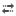to switch the variables on the x- and y-axis.

Use the Visualization type buttonto switch directly between a scatter plot and a summary table.

Click on the x- or y-axis to change the scale between Linear and Log.